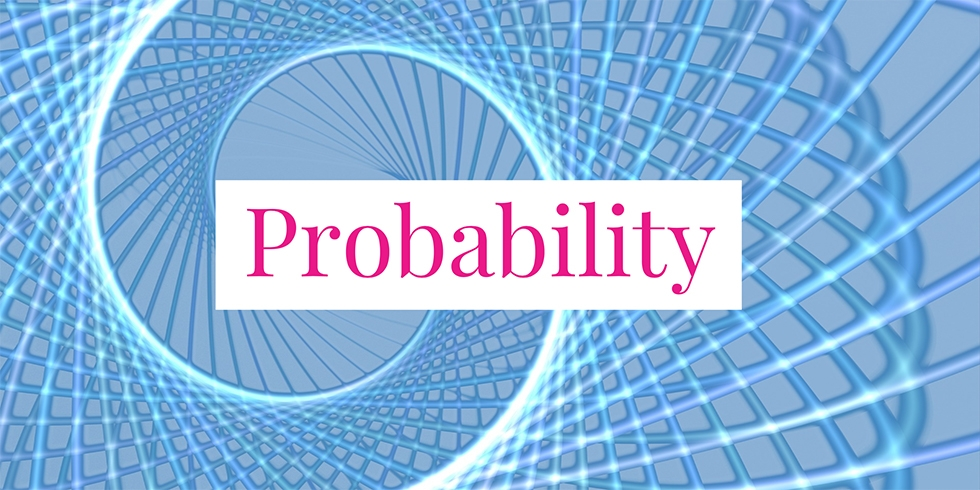# Jewelry Company Quality Test Failure Probability## Problem 731

A jewelry company requires for its products to pass three tests before they are sold at stores. For gold rings, 90 % passes the first test, 85 % passes the second test, and 80 % passes the third test. If a product fails any test, the product is thrown away and it will not take the subsequent tests. If a gold ring failed to pass one of the tests, what is the probability that it failed the second test?Add to solve later

## Solution.

Let $F$ be the event that a gold ring fails one of the three tests. Let $F_2$ be the event that it fails the second test. Then what we need to compute is the conditional probability
$P(F_2 \mid F) = \frac{P(F_2 \cap F)}{P(F)}.$

The numerator is
$P(F_2 \cap F) = P(F_2) = 0.9 \cdot 0.15.$ (A gold ring passes the first test with probability $0.9$ and fails the second test with probability $1-0.85=0.15$.)

The complement $F^c$ of $F$ is the event that a gold ring passes all the tests. Thus
$P(F) = 1- P(F^c) = 1 – 0.9 \cdot 0.85 \cdot 0.8.$ It follows that the desired probability is
\begin{align*}
P(F_2 \mid F) &= \frac{0.9 \cdot 0.15}{1 – 0.9 \cdot 0.85 \cdot 0.8}
= \frac{135}{388} \approx 0.348
\end{align*}

Therefore, given that a gold ring failed to pass one of the tests, the probability that it failed the second test is about 34.8 %.Add to solve later

### More from my site

#### You may also like...

This site uses Akismet to reduce spam. Learn how your comment data is processed.

###### More in Probability##### What is the Probability that All Coins Land Heads When Four Coins are Tossed If…?

Four fair coins are tossed. (1) What is the probability that all coins land heads? (2) What is the probability...

Close